Test: Continuity and Differentiability- Case Based Type Questions

# Test: Continuity and Differentiability- Case Based Type Questions

Test Description

## 10 Questions MCQ Test Mathematics (Maths) Class 12 | Test: Continuity and Differentiability- Case Based Type Questions

Test: Continuity and Differentiability- Case Based Type Questions for Commerce 2022 is part of Mathematics (Maths) Class 12 preparation. The Test: Continuity and Differentiability- Case Based Type Questions questions and answers have been prepared according to the Commerce exam syllabus.The Test: Continuity and Differentiability- Case Based Type Questions MCQs are made for Commerce 2022 Exam. Find important definitions, questions, notes, meanings, examples, exercises, MCQs and online tests for Test: Continuity and Differentiability- Case Based Type Questions below.
Solutions of Test: Continuity and Differentiability- Case Based Type Questions questions in English are available as part of our Mathematics (Maths) Class 12 for Commerce & Test: Continuity and Differentiability- Case Based Type Questions solutions in Hindi for Mathematics (Maths) Class 12 course. Download more important topics, notes, lectures and mock test series for Commerce Exam by signing up for free. Attempt Test: Continuity and Differentiability- Case Based Type Questions | 10 questions in 20 minutes | Mock test for Commerce preparation | Free important questions MCQ to study Mathematics (Maths) Class 12 for Commerce Exam | Download free PDF with solutions
 1 Crore+ students have signed up on EduRev. Have you?
Test: Continuity and Differentiability- Case Based Type Questions - Question 1

### Direction: Read the following text and answer the following questions on the basis of the same:A potter made a mud vessel, where the shape of the pot is based on f(x) = |x – 3| + |x – 2|, where f(x) represents the height of the pot.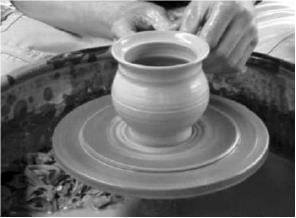If the potter is trying to make a pot using the function f(x) = [x], will he get a pot or not? Why?

Detailed Solution for Test: Continuity and Differentiability- Case Based Type Questions - Question 1 [x] is not continuous at integral values of x.
Test: Continuity and Differentiability- Case Based Type Questions - Question 2

### Direction: Read the following text and answer the following questions on the basis of the same:A potter made a mud vessel, where the shape of the pot is based on f(x) = |x – 3| + |x – 2|, where f(x) represents the height of the pot.Will the slope vary with x value?

Detailed Solution for Test: Continuity and Differentiability- Case Based Type Questions - Question 2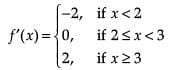Test: Continuity and Differentiability- Case Based Type Questions - Question 3

### Direction: Read the following text and answer the following questions on the basis of the same:A potter made a mud vessel, where the shape of the pot is based on f(x) = |x – 3| + |x – 2|, where f(x) represents the height of the pot.When the value of x lies between (2, 3) then the function is

Detailed Solution for Test: Continuity and Differentiability- Case Based Type Questions - Question 3 In (2, 3), f(x) = 1
Test: Continuity and Differentiability- Case Based Type Questions - Question 4

Direction: Read the following text and answer the following questions on the basis of the same:

A potter made a mud vessel, where the shape of the pot is based on f(x) = |x – 3| + |x – 2|, where f(x) represents the height of the pot.When x > 4 what will be the height in terms of x?

Detailed Solution for Test: Continuity and Differentiability- Case Based Type Questions - Question 4 The given function can be written as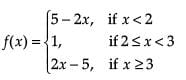When x > 4, f(x) = 2x – 5

Test: Continuity and Differentiability- Case Based Type Questions - Question 5

Direction: Read the following text and answer the following questions on the basis of the same:

A potter made a mud vessel, where the shape of the pot is based on f(x) = |x – 3| + |x – 2|, where f(x) represents the height of the pot.What is dy/dx at x = 3

Detailed Solution for Test: Continuity and Differentiability- Case Based Type Questions - Question 5 f(x) is not differentiable at x = 2 and x = 3.
Test: Continuity and Differentiability- Case Based Type Questions - Question 6

Direction: Read the following text and answer the following questions on the basis of the same:

Ms. Remka of city school is teaching chain rule to her students with the help of a flow-chart

The chain rule says that if h and g are functions and f(x) = g(h(x)), then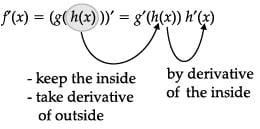Let f(x) = sin x and g(x) = x3

d/dx (sin2x) at x = π/2 is ____.

Detailed Solution for Test: Continuity and Differentiability- Case Based Type Questions - Question 6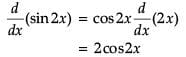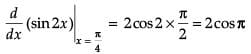= 2(-1)

= -2

Test: Continuity and Differentiability- Case Based Type Questions - Question 7

Direction: Read the following text and answer the following questions on the basis of the same:

Ms. Remka of city school is teaching chain rule to her students with the help of a flow-chart

The chain rule says that if h and g are functions and f(x) = g(h(x)), thenLet f(x) = sin x and g(x) = x3

gof(x) = _______.

Detailed Solution for Test: Continuity and Differentiability- Case Based Type Questions - Question 7 gof(x)

= g(f(x))

= g(sin x)

= sin3 x

Test: Continuity and Differentiability- Case Based Type Questions - Question 8

Direction: Read the following text and answer the following questions on the basis of the same:

Ms. Remka of city school is teaching chain rule to her students with the help of a flow-chart

The chain rule says that if h and g are functions and f(x) = g(h(x)), thenLet f(x) = sin x and g(x) = x3

d/dx sin x3 _______.

Detailed Solution for Test: Continuity and Differentiability- Case Based Type Questions - Question 8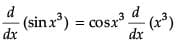= 3x2 cosx3

Test: Continuity and Differentiability- Case Based Type Questions - Question 9

Direction: Read the following text and answer the following questions on the basis of the same:

Ms. Remka of city school is teaching chain rule to her students with the help of a flow-chart

The chain rule says that if h and g are functions and f(x) = g(h(x)), thenLet f(x) = sin x and g(x) = x3

fog(x) = _______.

Detailed Solution for Test: Continuity and Differentiability- Case Based Type Questions - Question 9 fog(x)

= f(g(x))

= f(x3)

= sin (x3)

Test: Continuity and Differentiability- Case Based Type Questions - Question 10

Direction: Read the following text and answer the following questions on the basis of the same:

Ms. Remka of city school is teaching chain rule to her students with the help of a flow-chart

The chain rule says that if h and g are functions and f(x) = g(h(x)), thenLet f(x) = sin x and g(x) = x3

d/dx (sin3 x) = _______.

Detailed Solution for Test: Continuity and Differentiability- Case Based Type Questions - Question 10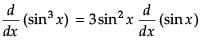= 3 sin2 x cos x

## Mathematics (Maths) Class 12

209 videos|209 docs|139 tests
 Use Code STAYHOME200 and get INR 200 additional OFF Use Coupon Code
Information about Test: Continuity and Differentiability- Case Based Type Questions Page
In this test you can find the Exam questions for Test: Continuity and Differentiability- Case Based Type Questions solved & explained in the simplest way possible. Besides giving Questions and answers for Test: Continuity and Differentiability- Case Based Type Questions, EduRev gives you an ample number of Online tests for practice

## Mathematics (Maths) Class 12

209 videos|209 docs|139 tests